# HOW  TO   TEST  ZENER  DIODE   by   digital multimeter   and  Analog multimeterZENER  DIODE TESTING

ZENER  DIODE TESTING    Zener diode is a subtype  of  a diode  and  it  use on circuit as   a different function from standard diode.   Standard diode we use it as forward bias mode but  for Zener diode we use it as reverse  bias mode on circuit.  When  applying  forward bias to  zener diode it will conduct and let current flow as standard diode  ,  another condition  when  applying  reverse bias to zener diode it will not let current flow until   the  voltage supply  is  over  the zener  breakdown voltage then it will let current flow.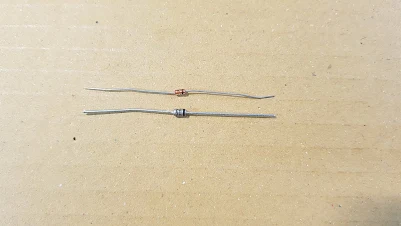ZENERBasic  Circuit  of   zener DiodeBasic  Circuit  of   zener Diode

How to check zener diode , it has more detail then checking standard diode  as it is  a subtype of diode so in some condition  checking method  is  as  same as standard diode.   Before checking you have to know  important technical detail of multimeter.  We  set  analog multimeter to  range Rx1 or  Rx10 , let consider at the test lead there is 3VDC 150mA for  Rx1 range  and  3VDC 15mA for Rx10.There is  3VDC 150mA  at  the test leads ,   Rx1 range,  of  Analog Multimeter.There is 3VDC 15mA  at the test  leads ,  Rx10  range,  of  Analog Multimeter.There is 3.28VDC  at the test leads  of   Diode Test  Function of  Digital mulimeter(some model)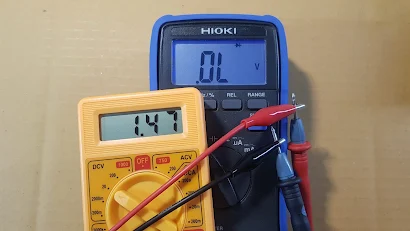There is 1.47VDC  at the test leads  of  Diode Test  Function of  Digital mulimeter(some model)

Step to check Zener Diode with  Digital multimeter.
1.  Turn rotary switch  of   digital multimeter to  Diode Function test.
2.  If  zener diode has  zener  breakdown voltage above the lead test voltage .
( We use  Digital mulimeter that  has  3.28VDC at test leads)
2.1  If it is a  good zener Diode it will show voltage drop across diode when applying  forward bias.
and  show "OL" when applying reverse bias.
2.2  If it is a  BAD zener Diode ( Open) , it show " OL"  on both forward bias and  reverse bias.
2.3  If it is a  BAD zener Diode  ( shorted ) ,  it show very small voltage drop  on both forward bias and  reverse bias.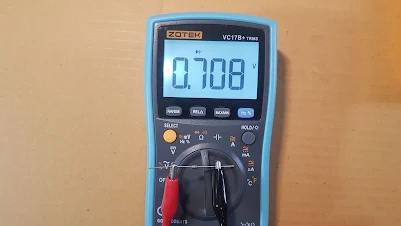There is  voltage drop across  0.4-0.7V  when appply  forward bias ( for a good  Zener  diode)" OL"  display  when  applying   reverse  bias ( If it's a good  zener  diode).Very low voltage  drop  when it is a  shored Zener Diode

3.  If  a   zener diode has  zener  breakdown voltage  below  the lead tests.  we use  Digital mulimeter that  has  3.28VDC at test leads.
3.1  When applying forward bias to  zener diode it  will let current flow and there is voltage drop across it  around  0.4-0.7V  and  when  applying reverse bias to zener diode it  will let current flow too as  voltage magnitude  from the test leads  is over   zener  breakdown voltage , for example 2.7V zener diode , it will display  2.7V when applying reverse bias.
3.2  If it is a  BAD zener Diode (open) , it show " OL" on both forward bias and  reverse bias.
3.3 If it is a  BAD  zener Diode (shorted) ,  it show very small voltage drop  on both forward bias and  reverse bias ( such as  0.002V).

Step  to c heck  Zener  Diode  with  Analog  multimeter.
1.  Turn the rotary switch of    analog  multimeter to  Rx1  test    Function.
2.  If  a  zener diode has  zener  breakdown voltage above the  test leads  voltage.  We use  Analog  mulimeter that  has  3.1VDC  at  it's  test leads.
2.1  If it is a  good zener Diode it will show low resistance  around 3-10  ohm when applying forward bias.  and   pointer point at   ∞    resistance  scale  when applying  reverse  bias.
2.2  If it is a BAD  zener Diode ( open)  , pointer point at     Infinity  resistance for  both forward bias and  reverse bias.
2.3 If it is a  BAD  zener Diode (shorted )  it show  0 ohm resistance for  both forward bias and  reverse bias.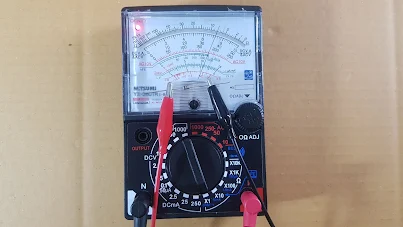Good  Zener Diode  has  low resistance  around 3-10  ohm when applying  forward bias.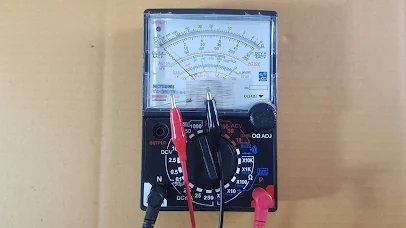Good  Zener Diode  has  ∞  infinity  resistance  when applying  reverse   bias.Shorted  zener Diode has  0 ohm resistance  for  both forward bias and  reverse bias.

3.  If  a   zener diode has  zener  breakdown voltage  below  the test  leads  voltage (for example ZD = 2.7V) .  We use  analog  mulimeter that  has  3.1VDC  at  it's  test leads.
3.1 when  applying  forward bias to  zener diode it  will let current flow and there is  low resistance around 3-10 ohm  and  when reverse bias to zener diode it  will let current flow too as  the voltage at test leads  is over zener break down voltage  so current flow and there is some low resistance ( but resistance is not as low as shorted case 0 ohm)
3.2  If it is a   BAD   zener Diode (open ) ,  pointer point at    infinity  resistance scale  for both forward  bias  and reverse   bias.

3.3 If it is a BAD  zener Diode (shorted) ,  pointer point at  0 ohm  resistance  for  both forward  bias  and reverse   bias.